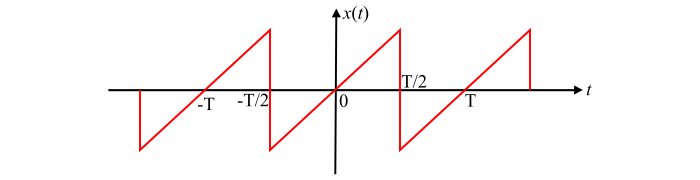# Signals and Systems – What is Half Wave Symmetry?

## Importance of Wave Symmetry

If a periodic signal $x(t)$ has some type of symmetry, then some of the trigonometric Fourier series coefficients may become zero and hence the calculation of the coefficients becomes simple.

## Half Wave Symmetry

A periodic function $x(t)$ is said to have half wave symmetry, if it satisfies the following condition −

$$\mathrm{x(t)=-x\left ( t ± \frac{T}{2}\right )… (1)}$$

Where, $T$ is the time period of the function.A periodic function $x(t)$ having half wave symmetry is shown in the figure. It can be seen that this function is neither purely even nor purely odd. For such type of functions, the DC component of Fourier expansion is zero, i.e.,$a_{0}=0$ Also, the Fourier expansion of such functions contains odd harmonic components only, that is $\omega_{0},3\omega_{0},5\omega_{0},...$ etc.

Since the periodic function $x(t)$ having half wave symmetry contains only odd harmonic terms. Therefore, when n is even $a_{n}=b_{n}=0$.

And when n is odd, then, the coefficients $a_{n}$ and $b_{n}$ can be determined as follows −

$$\mathrm{a_{n}=\frac{2}{T}\int_{0}^{T}x(t)cos\:n\omega_{0}t\:dt}$$

$$\mathrm{\Rightarrow\:a_{n}=\frac{2}{T}\left [ \int_{0}^{T/2} x(t)cos\:n\omega_{0}t\:dt+\int_{T/2}^{T} x(t)cos\:n\omega_{0}t\:dt\right ]}$$

Replacing the variable from $t$ to $(t+\frac{T}{2})$ in the second integral term, we have,

$$\mathrm{a_{n}=\frac{2}{T}\left [ \int_{0}^{T/2} x(t)cos\:n\omega_{0}t\:dt+ \int_{0}^{T/2} x(t+\frac{T}{2})cos\:n\omega_{0}(t+\frac{T}{2})dt\right ]}$$

By the definition of half wave symmetry, i.e.,$x(t)=-x(t ± \frac{T}{2})$ , we have,

$$\mathrm{a_{n}=\frac{2}{T}\int_{0}^{T/2}[x(t)\:cos\:n\omega_{0}t-x(t)\:cos\:n\omega_{0}t\:cos\:n\pi]dt… (2)}$$

Therefore, the Fourier series coefficient $a_{n}$ for a function having half wave symmetry can be defined as,

$$\mathrm{a_{n}=\begin{cases}0; & for\:even\:n\\frac{4}{T}\int_{0}^{T/2}\:x(t)\:cos\:n\omega_{0}t\:dt\:;& for\:odd\:n\end{cases}}$$

Similarly, the coefficient $b_{n}$ is given by,

$$\mathrm{b_{n}=\begin{cases}0; & for\:even\:n\\frac{4}{T}\int_{0}^{T/2}\:x(t)\:sin\:n\omega_{0}t\:dt\:;& for\:odd\:n\end{cases}}$$